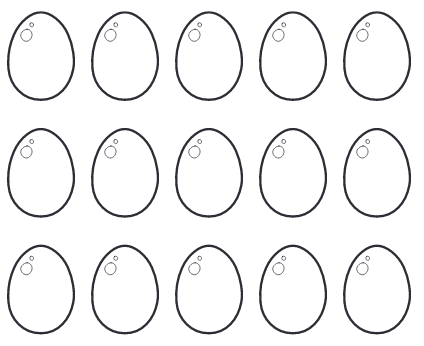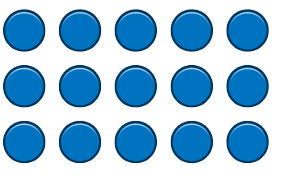# Mathematical RepresentationsOne of the 5 Big Ideas of mastery is Representation and Structure. A focus on exposing the structure of mathematical and developing an understanding of how and why maths works is crucial to mastery.

Concrete materials, contexts, drawings, diagrams and equations all play a role. These are discussed through opportunities for pupil-pupil and pupil-teacher talk, to develop reasoning, flexibility and adaptability in mathematical thinking.

The images below show how concrete objects, drawings, diagrams and equations used in the teaching and learning of additio, subtraction, multiplication and division. The same representations are used when teaching the four operations, which enables children to make connections between the concepts they have mastered. The same representations are used across the year groups.Multiplication and Division

Arrays

Arrays are a visual representation of multiplication and division. They are arranged into rows and columns, clearly showing equal groups within a number. This helps children to distinguish between each number in multiplication and division calculations. They are an excellent tool for showing what X groups of Y is equivalent to. They also clearly show the commutativity of multiplication (i.e. how ‘X groups of Y ’ has the same total as ‘Y groups of X’).5 + 5 + 5 = 15                       3 + 3 + 3 + 3 + 3 = 15

3 groups of 5 = 15                5 groups of 3 = 15

3 x 5 = 15                             5 x 3 = 1515 shared into 3 equal parts. There are 5 in each part.

15 ÷ 3 = 5

15 divided into groups of 5. There are 3 groups.

15 ÷ 5 = 3